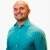# SAT Prep How to determine the probability of a situationThis lesson will look at an example problem involving probability. This lesson will give an explanation of how to determine the probability of a situation with a step by step look to better explain the concept.
9 Views